Russian Academy of Sciences Central Astronomical Observatory at Pulkovo SIXTH US/RUSSIAN SPACE SURVEILLANCE WORKSHOP August 22-26, 2005 Proceedings Edited by P. Kenneth Seidelmann and Victor K. Abalakin St. Petersburg, 2005<<< Contents

### NONLINEAR BAYESIAN FILTERING FOR STATE AND PARAMETER ESTIMATION

Kyle T. Alfriend* and Deok-Jin Lee**

Texas A&M University, College Station, TX, 77843-3141

Abstract. This paper provides efficient filtering algorithms for nonlinear estimation. Adaptive nonlinear filtering for recursive estimation of the states and parameters with unknown noise statistics and a novel method called the sigma particle filter for nonlinear/non-Gaussian estimation are illustrated. The adaptive nonlinear filters combine adaptive estimation techniques for system noise statistics with nonlinear filters that include the unscented Kalman filter and divided difference filter. The purpose of the new nonlinear filters is to not only compensate for the nonlinearity effects neglected from linearization by utilizing nonlinear filters, but also to take into account the system modeling errors by adaptively estimating the noise statistics and unknown parameters. Simulation results indicate that the advantages of the nonlinear filters make these attractive alternatives to the standard nonlinear filters for the state and unknown parameter estimation in not only satellite orbit determination but also other application areas.
________________________
* TEES Distinguished Research Chair Professor, Department of Aerospace Engineering, Texas A&M University, College Station, TX 77843-3141
** Postdoctoral Research Associate, Department of Aerospace Engineering, Texas A&M University, College Station, TX 77843-3141
##### I. INTRODUCTION

The nonlinear filtering problem consists of estimating the states of a nonlinear stochastic dynamical system. The class of systems considered is broad and includes orbit/attitude estimation, integrated navigation, and radar or sonar surveillance systems1. Because most of these systems are nonlinear and/or non-Gaussian, a significant challenge to engineers and scientists is to find efficient methods for on-line, real-time estimation and prediction of the dynamical systems and error statistics from the sequential observations. In a broad sense, general approaches to optimal nonlinear filtering can be described in a unified way using the recursive Bayesian approach2~4. The central idea of this recursive Bayesian estimation is to determine the probability density function of the state vector of the nonlinear system conditioned on the available measurements. This a posterior density function provides the most complete description of an estimate of the system. In linear systems with Gaussian process and measurement noises, an optimal closed-form solution is the well-known Kalman filter5. In nonlinear systems the optimal exact solution to the recursive Bayesian filtering problem is intractable since it requires infinite dimensional processes6. Therefore, approximate nonlinear filters have been proposed. These approximate nonlinear filters can be categorized into five types: (1) analytical approximations, (2) direct numerical approximations, (3) sampling-based approaches, (4) Gaussian mixture filters, and (5) simulation-based filters. The most widely used approximate nonlinear filter is the extended Kalman filter, which is the representative analytical approximate nonlinear filter. However, it has the disadvantage that the covariance propagation and update are analytically linearized up to the first-order in the Taylor series expansion, and this suggests that the region of stability may be small since nonlinearities in the system dynamics are not fully accounted for7. Thus, the purpose of this research is to investigate new and more sophisticated nonlinear estimation algorithms, develop new nonlinear filters, and demonstrate their applications in accurate spacecraft orbit estimation and navigation.

The work presented here involves the investigation of system identification and nonlinear filtering algorithms that are compatible with the general goals of precise estimation and autonomous navigation. In this paper, efficient alternatives to the extended Kalman filter (EKF) are suggested for the recursive nonlinear estimation of the states and parameters of aerospace vehicles. First, approximate (suboptimal) nonlinear filtering algorithms, called sigma point filters (SPFs) that include the unscented Kalman filter (UKF)8, and the divided difference filter (DDF)9, are reviewed. The unscented Kalman filter, which belongs to a type of sampling-based filters, is based on the nonlinear transformation called the unscented transformation in which a set of sampled sigma points are used to parameterize the mean and covariance of a probability distribution efficiently. The divided difference filter, which falls into the sampling-based polynomial filters, adopts an alternative linearization method called a central difference approximation in which derivatives are replaced by functional evaluations, leading to an easy expansion of the nonlinear functions to higher-order terms. Secondly, a direct numerical nonlinear filter called the finite difference filter (FDF) is introduced where the state conditional probability density is calculated by applying fast numerical solvers to the Fokker-Planck equation in continuous-discrete system models10. However, most of the presented nonlinear filtering methods (EKF, UKF, and DDF), which are based on local linearization of the nonlinear system equations or local approximation of the probability density of the state variables, have not been universally effective algorithms for dealing with both nonlinear and non-Gaussian system.

For these nonlinear and/or non-Gaussian filtering problems, the sequential Monte Carlo method is investigated11~14. The sequential Monte Carlo filter can be loosely defined as a simulation-based method that uses a Monte Carlo simulation scheme in order to solve on-line estimation and prediction problems. The sequential Monte Carlo approach is known as the bootstrap filtering11, and the particle filtering14. The flexible nature of the Monte Carlo simulations results in these methods often being more adaptive to some features of the complex systems15. There have been many recent modifications and improvements on the particle filter15. However, some of the problems, which are related to the choice of the proposal distribution, optimal sampling from the distribution, and computational complexity, still remain. This work investigates a number of improvements for particle filters that are developed independently in various engineering fields. Furthermore, a new type of particle filter called the sigma particle filter16 is proposed by integrating the divided difference filter with a particle filtering framework, leading to the divided difference particle filter. The performance of the proposed nonlinear filters is degraded when the first and second moment statistics of the observational and system noise are not correctly specified17,18. Sub-optimality of the approximate nonlinear filters due to unknown system uncertainties and/or noise statistics can be compensated by using an adaptive filtering method that estimates both the state and system error statistics19,20.

Two simulation examples are used to test the performance of the new nonlinear filters. First, the adaptive nonlinear filtering algorithms with applications to spacecraft orbit estimation and prediction are investigated and then the performance of the new sigma particle filter is demonstrated through a highly nonlinear falling body system.
##### II. OPTIMAL BAYESIAN ESTIMATION

Sequential Monte Carlo (SMC) filtering is a simulation of the recursive Bayes update equations using a set of samples and weights to describe the underlying probability distributions11. The starting point is the recursive Bayes observation update equation, which is given by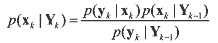(1)

The Chapman-Kolmogorov prediction equation is introduced by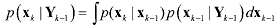(2)

where xk is the state of interest at time k, yk is an observation taken of this state at time k, and Yk ≡ {y1,..., yk} is the set of all observations up to time k. Eqs. (1) and (2) define a set of recursive equations for the calculation of the posterior p(xk|Yk-1) in terms of an observation model or likelihood function p(yk|xk) and a process model or transition probability p(xk|xk-1) .

For most applications, however, closed-form solutions of the posterior update equation p(xk|Yk) are not tractable due to the multiple integrations in the prediction equation. Depending on the characteristics of the system, there exist different methods of estimating the posterior density p(xk|Yk). In a broad sense there are three different cases of filtering, namely linear Gaussian, nonlinear Gaussian, and nonlinear/Non-Gaussian. First, for a linear- Gaussian case where the system dynamic and measurement equations are linear, and the a priori initial state and the noise sequences are Gaussian, the recursive Bayesian estimation in Eqs. (1) and (2) leads to the Kalman filtering algorithm. Second, if the system equations are nonlinear with the assumption of Gaussian distributions, the Gaussian filters such as EKF, UKF, and DDF provide the sub-optimal recursive algorithms. Finally, for nonlinear and non- Gaussian problems, the optimal Bayesian equations are solved by sequential Monte-Carlo methods. In a broad sense, the unscented Kalman filter belongs to the particle filters in that it performs sequential estimation based on a set of sampled particles drawn deterministically from probability densities. The difference is that the sigma point filters (UKF, DDF) make a Gaussian assumption to simplify the recursive Bayesian estimation, whereas the particle filter performs estimation based on the form of general nonlinear models and non-Gaussian distributions. In the next section, the particle filter also called the bootstrap filter is illustrated.
##### III. SUBOPTIMAL NONLINEAR FILTERING

This section illustrates the integration of the proposed adaptive filtering algorithms with the sigma point filters (SPFs)21, such as UKF and DDF, for more enhanced nonlinear filtering algorithms. Thus, the adaptive sigma point filters (ASPFs) lead to the adaptive unscented Kalman filter (AUKF) and the adaptive divided difference filter (ADDF). The objectives of the integrated adaptive nonlinear filters are to take into account the incorrect time-varying noise statistics of the dynamic systems and to compensate for the nonlinearity effects neglected by linearization.
##### 1. Adaptive Unscented Kalman Filtering

The unscented Kalman filtering8 algorithm is summarized for discrete-time nonlinear equations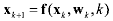(3)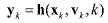(4)

where xk Є Rn is the n×1 state vector and yk Є Rm is the m×1 measurement vector at time k. wk Є Rq is the q×1 process noise vector and vk Є Rк is the r×1 additive measurement noise vector, and they are assumed to be zero-mean Gaussian noise processes with unknown covariances given by Qk and Rk respectively. The original state vector is redefined as an augmented state vector xak along with noise variables, and an augmented covariance matrix Pak on the diagonal is reconstructed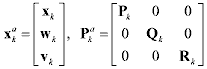(5)

where na = n + q + r is the dimension of the augmented state vector. Then, a set of the scaled symmetric sigma points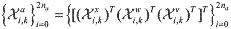is constructed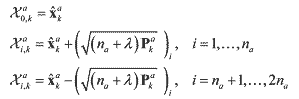(6)

where λ = α2(na + κ) - na includes scaling parameters. α controls the size of the sigma point distribution and should be a small number (0 ≤ α ≤ 1) and κ provides an extra degree of freedom to fine tune the higher order moments κ = 3 - na for a Gaussian distribution.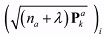is the ith column or row vector of the weighted square root of the scaled covariance matrix (na + λ)Pak.

As for the state propagation step, the predicted state vector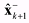and its predicted covariance P-k+1 are computed using the propagated sigma point vectors.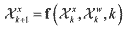(7)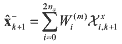(8)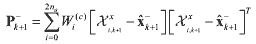(9)

where Χxi,k is a sigma point vector of the first n elements of the ith augmented sigma point vector Χai,k and Χwi,k is a sigma point vector of the next q elements of ith augmented sigma point vector Χai,k, respectively. Similarly, the predicted observation vector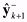and the innovation covariance Pυυk+1 are calculated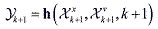(10)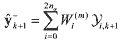(11)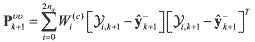(12)

where Χνi,k is a sigma point vector in the r elements of the ith augmented sigma point vector Χai,k. Wi(m) is the weight for the mean and Wi(c) is the weight for the covariance given by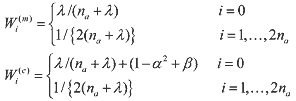(13)

where β is a third parameter that further incorporates higher order effects by adding the weighting of the zeroth sigma point of the calculation of the covariance, and β = 2 is the optimal value for Gaussian distributions. Now, the Kalman gain Κ(k+1) is computed by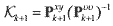(14)

and the cross correlation matrix is determined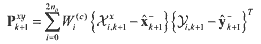(15)

The estimated state vector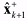and updated covariance P+k+1 are given by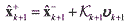(16)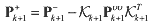(17)

where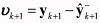is the innovation vector that is the difference between the observed measurement vector and the predicted measurement vector.

Note that for implementing the proposed adaptation algorithm into the SPFs the expression of the process noise covariance matrix in the predicted covariance equation should be explicit. However, the process noise covariance term in the UKF algorithm is implicitly expressed in the predicted covariance equation, thus, the noise adaptive estimator cannot be directly implemented.

There are two approaches that can integrate the proposed adaptive algorithm into the unscented Kalman filtering21. The first method is to use the augmented covariance matrix Pak given in Eq. (15), where the current process covariance matrix Qk is replaced with the estimated covariance Q^k and at each time step new sigma points drawn from the new augmented covariance matrix P^ak are used for the propagation step.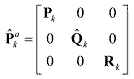(18)

The second approach uses the assumption that both the process and measurement noises are purely additive. Then, the sigma point vectors Χwk and Χνk for the process and measurement noise are not necessary, and the sigma point vector reduces to Χak = ΧxkΧk. Thus, the process noise covariance can be expressed explicitly in the predicted covariance equation as(19)

Now, the noise adaptation estimator can be directly applied to formulate an adaptive unscented Kalman filter algorithms. From Maybeck’s unbiased adaptation algorithm22, the observation of the process noise matrix was rewritten as the difference between the state estimate before and after the measurement update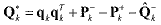(20)

where the term qkΚkvk is the state residual and represents the difference between the state estimates before and after the measurement update. Q^-kQk is the current expected process noise covariance. If the residual has a large value, then it indicates that the future state prediction is not accurate enough. The first term in the above equation is a measure of the state residual, and the next term is a measure of the correction in the expected change of covariance. It is rewritten and becomes obvious conceptually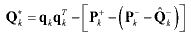(21)

The equation shows that Qk is the residual minus the change in the a posteriori covariances between two consecutive time steps.20 The measure of the process noise Qk is then combined with the current estimate Q^-k in a moving average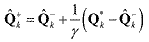(22)

where γ is the window size that controls the level of expected update change and needs to be selected through a trial-error method. If γ is small, then each update is weighted heavily, but if γ is large, then each update has a small effect. The performance of the adaptive routine is very sensitive to the selection of γ, and thus should be selected for each application. Denote QkQ^+k, then the estimated covariance matrix is used in the covariance propagation step in Eq. (18). Now, the discrete formulation is placed into a continuous form. If the estimated discrete-time process noise covariance matrix is written by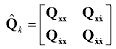(23)

then diagonalization of the process noise covariance of the velocity part can be made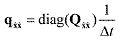(24)

Now the continuous process noise covariance update is redefined as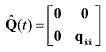(25)

This updated estimate Q^(t) is used for the state propagation between time-step t and t+dt.

Note that the proposed adaptive algorithms highly depend on the selection of the weight factor γ. In order to provide the consistent, optimal performance of the proposed adaptive filter, we suggest an efficient calibration method in this paper. Now the question comes to mind is how the scaling factor γ should be determined. The brute-force way for computing the scale factor is to use the trial-error approach until the filter produces a sub-optimal or near-optimal estimation result. In this paper, an efficient derivative-free numerical optimization technique is utilized for automated calibration of the weight scale factor. The numerical optimization method called the Downhill Simplex algorithm21 is used to tune the parameters of the process noise covariance. In order to apply the numerical optimization algorithm to the filter tuning, the tuning problem must be expressed by a numerical optimization or function minimization problem. The objective function is constructed in terms of the innovation vector concept instead of the estimate error. To obtain an on-line optimal estimator it is assumed that the system noise was slowly varying over Ns time steps. The cost function is expressed as follows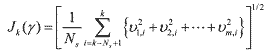(26)

where υm,i is each component of the innovation vector, Ns is the number of time steps, and m is the dimension of the observation vector. The objective of the optimization is to find a optimal weight parameter γopt such that it minimizes the sum of the innovation errors or the state errors. The weight scale factor can also be obtained off-line by making use of the cost function including all the innovation errors over the entire estimation period.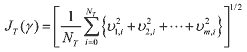(27)

where NT is the total number of time steps over the entire estimation period. Note that in the off-line method if the initial innovation vectors contain large errors it is necessary to neglect them in the cost function because large initial innovation errors make the estimator converge slowly.
##### 2. Adaptive Divided Difference Filtering

In this section, the proposed noise estimator algorithm is combined with the divided difference filter (DDF)9 such that the integrated filtering algorithm leads to the adaptive divided difference filter (ADDF). The first-order divided difference filter (DDF1) is illustrated for general discrete-time nonlinear equations with the assumption that the noise vectors are uncorrelated white Gaussian processes with unknown expected means and covariances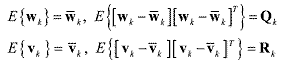(28)

First, the square Cholesky factorizations are introduced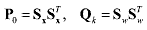(29)

The predicted state vector x^-k+1 and the predicted state covariance P-k+1 are computed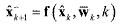(30)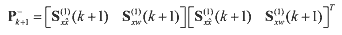(31)

where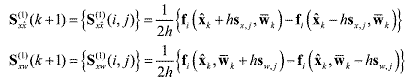(32)

where sx,j is the column of Sx and sw,j is the column of Sw from Eq. (29) . Next, the square Cholesky factorizations are performed again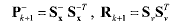(33)

The predicted observation vector y^-k+1 and its predicted covariance are calculated in a similar fashion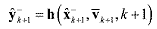(34)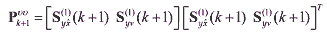(35)

where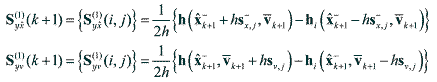(36)

where s-x,j is the column of S-x and sv,j is the column of Sv. If the measurement noise vector is simply additive, then the innovation covariance Pυυk+1 is computed as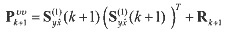(37)

Finally the cross correlation matrix is determined by using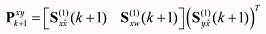(38)

In the update process, the filter gain Κk+1, the estimated state vector x^+k+1, and updated covariance P+k+1 can be computed by using the same formulas as in the UKF.

For the adaptive divided difference filter formulation, the method used to combine the proposed noise estimator with the DDF is to just perform the square Cholesky factorization sequentially at each time when the estimated covariance is updated from the noise adaptation. If the estimated covariance matrix in Eq. (22) is factorized at each time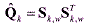(39)

then, the factorized value is delivered back to the DDF algorithm leading to adaptive filtering structure.
##### 3. Sigma Particle Filtering

In this section a new particle filtering method, which is based on the bootstrap filter11 and a new sigma particle sampling method, is illustrated. The new particle filter is called the sigma particle filter. In this section, a specific algorithm for the sigma particle filter (SPF) is explained. The implementation of the sigma particle filter consists of three important operations; 1) prediction of sigma particles, 2) update of the particle weights with measurements, and 3) drawing sigma particles.
##### A. Initial Sampling

There are two different ways in which initial sigma particles are drawn23. In this paper, the first method, called the simplified sigma particle sampling method (SSPS), is illustrated. The particle sampling method yields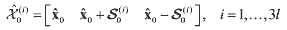(40)

where x^0 is the initial estimate. The second option is to use the random sampling used in the particle filtering from either a Gaussian distribution or uniform distribution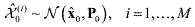(41)

where M is a larger number than that of N in the sigma particles, (M >> N). Even though M random samples are used in the prediction at the next step, the number of samples is reduced to N in the update procedure by adopting the sigma particle sampling method.
##### B. Prediction

The prediction of the drawn samples is performed by using the system dynamic function in the same way as the particle filtering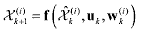(42)

where w(i)k are the N samples of the process noise drawn by w(i)k ~ p(wk).
##### C. Update

The important weights are updated by selecting the important function as the state propagation density q(xk+1|Xk, Yk+1)=q(xk+1|xk), which yields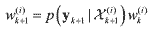(43)

where the likelihood probability density p(yk+1|Χ(i)k+1) is computed by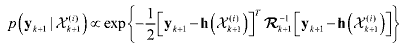(44)

and Rk+1 is an exponentially time-varying measurement noise covariance matrix, which plays an important role of adjusting the variance of the likelihood function. The idea of this time- varying measurement covariance matrix is to adjust the likelihood function so that particles with high important weights can be produced. Let the initial measurement covariance matrix Rk be multiplied by a scale factor λs, and denote R0=λsRk. Then, the exponentially time- varying measurement noise covariance Rk+1 is computed by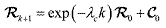(45)

where λc is a time constant controlling the decaying rate, λs is a scale factor adjusting the size of the measurement noise variance, C0 is a constant tuning matrix, and k is the discrete-time index. Note that it is necessary to tune the constant parameters to produce the best estimate results. If necessary, the total simulation period can be divided into sections, and a different exponentially time-varying measurement covariance matrix Rk+1 can be applied to each corresponding section. The updated weights are normalized for the next time step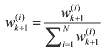(46)

Now, an estimate of the current mean value and covariance is obtained by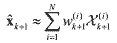(47)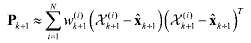(48)

where w(i)k+1 are the normalized weights in the update step, and the state samples x(i)k+1 are obtained in the prediction step. Now, it is necessary to update the sigma particles from the estimated mean and covariance by using either the GSPS or SSPS method23. Applying the SSPS method yields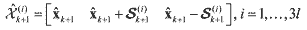(49)

Then, newly sampled particles are fed back to the prediction step.
##### SIMULATION RESULTS

The criteria for judging the performance of the proposed adaptive filters are the magnitude of the residuals and their statistics. If the measurement residuals or the state estimation errors are sufficiently small and consistent with their statistics, then the filter is trusted to be operating consistently. In other words, the most common way for testing the consistency of the filtering results is to depict the estimated state errors with the 3-sigma bound given by. If the errors lie within the bound, the estimation result is believed to be consistent and reliable. Instead of the state estimation errors, the measurement innovation vector can be also used for the filter performance analysis. If the measurement residuals lie within the 2-sigma bound,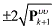, it indicates the 95% confidence of the estimation results.
##### 1. Orbit Estimation Example

The performance of the proposed adaptive nonlinear filters, the AEKF, AUKF, and ADDF is demonstrated through simulation examples using realistic satellite and observation models. The satellite under consideration has the following orbit parameters: a = 6978.136km, e = 1.0×10-5, i = 51.6°, ω = 30°, Ω = 25°, E = 6°. The system dynamic equations consist of the two-body motion, J2 zonal perturbation and drag perturbation. The ballistic parameter taken is B* = 1.0×106 kg/km2 where the drag coefficient has Cd = 2.0. All dynamic and matrix differential equations are numerically integrated by using a fourth-order Runge-Kutta algorithm.

One ground tracking site was used to take observations and the location of the sensor was selected to be Eglin Air Force Base whose location is at 30.2316° latitude and 86.2147° longitude. Each observation consists of range, azimuth and elevation angles, and the measurement errors are considered to be Gaussian random processes with zero means and variances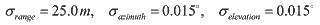(50)

Observations are available from two widely separated tracks. Thus the estimated state and covariance matrix from the first track are propagated up to the next available measurements. The second track has some uncertainty as well as nonlinearity due to the propagation from the end of the first track. Each track length is 2~3 minutes with observations at every 5 seconds. Visibility of the satellite is decided by checking the elevation angle (EL). If EL is less than 10°, prediction is performed until the next available observation with EL greater than 10°. Once EL crosses the threshold boundary, estimation begins and the estimation mode continue during the 2~3 minutes.

The state vector for the UKF is augmented with the process noise terms wk,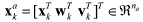. The parameters used in the UKF are the scaling factors associated with the scaled unscented transformation. β = 2 is set to capture the higher order (fourth) terms in the Taylor series expansion, κ = 3 - nα is used for multi-dimensional systems, and α = 10-3 is chosen to be small in order to make the sample distance independent of the state size. The interval length h = 3 is set for a Gaussian distribution in the DDF.
##### First Track Estimation

The “solve-for” state vector xk consists of the position, velocity, and drag coefficient, x = [x,y,z,x,y,z,Cd]T . The true initial values of the state variables were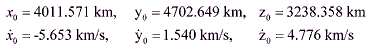(51)

and the drag coefficient was Cd = 2.0. For the nominal reference trajectory, the following initial estimates were used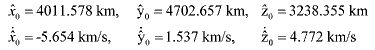(52)

and the initial estimate of the drag coefficient was C^d = 3.0. The initial covariance P0 є R7×7 used for the filters had diagonal elements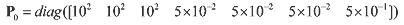(53)

Often, the process noise for the dynamic model errors is added to the acceleration terms to help adjust the convergence properties. In this study, however, the value for Q(t) is set, rather than adjusted, in order to model the realistic environment as close as possible. For instance, the acceleration due to J2 is approximately 10-5 km/sec2 , and the truncated or ignored perturbing accelerations are roughly of order J22. Therefore, in the orbit model, the process noise matrix takes the values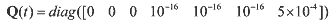(54)

Note that since the process noise covariance Q(t) comes from a continuous-time dynamic system model, it is necessary to convert it into the discrete-time form of the covariance Qk through an approximate numerical integration scheme1, that is, QkQ(tt, where Δt is the time step.

For establishing accurate estimation conditions a few measurements were utilized to produce an initial orbit determination of the state of the satellite. The initial state estimation was executed by employing the Herrick-Gibbs algorithm24 as a batch filter such that it is assumed that the conditions for sub-optimality or near-optimality of the filters are satisfied in the first track. Therefore, in the first track the performance of the three nonlinear filters EKF, UKF, and DDF are compared without integration of the nonlinear adaptive estimator.

The absolute magnitude values of the position and velocity errors for the three nonlinear filters are shown in Figures 1 and 2, respectively. As can be seen, the advantage of the SPFs over the EKF in the position and velocity estimation errors is not obvious, but the SPFs are slightly better, which indicates that the effect of nonlinearity on the filters is not severe with the small initial state errors along with the small process noises over the short track length.

Fig. 3 shows the measurement innovation errors that lie inside the 2-sigma bound without any deviations. Even though each filter produces slightly different innovation errors, they all fall inside the boundary with close optimal performance. According to the criteria such as the RMS errors, and the innovation error, it is seen that the performance of the nonlinear filters is near-optimal when the process and measurement noise are correctly selected but the SPFs are slightly better than the conventional EKF due to the neglected nonlinear effect.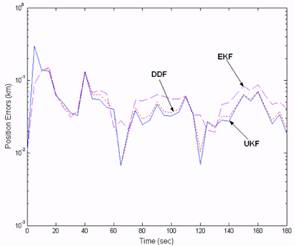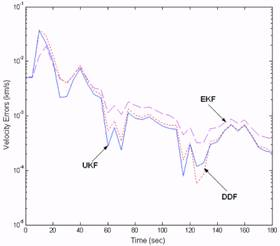Fig. 1. Absolute Position Estimation Errors in First Track. Fig. 2. Absolute Velocity Estimation Errors in First Track.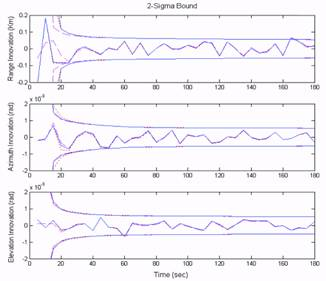Fig. 3. Measurement Innovation Errors with 2-Sigma Bound.
##### Second Track Estimation

The second track was separated from the first track by approximately 24 hours. Due to the long propagation time large state errors occur. During the prediction period between the first and second tracks, each propagation method in the filters was used in order to propagate the estimated state and covariance matrix. Thus, the inputs to the orbit determination in the second track are the a priori state estimate and covariance matrix propagated from the end of the first track, and each nonlinear filter has the same initial state and covariance values. The prediction equations used consist of the two-body motion, J2 zonal perturbation and drag perturbation, but no process noise was added on it.

There are two factors that affect the accuracy of the second track estimation, the separation time and the first track length. As the separation time between tracks increases the prediction errors increase due to the neglected nonlinear terms in the prediction process, which affects the estimation in the second track. Also, large errors could occur even if the system was linear because a 3-minute 1st track does not allow an accurate state estimate. The uncertainties or neglected modeling errors in the second track, however, can be compensated for by utilizing the proposed adaptive nonlinear filtering techniques that adaptively estimate the process noise covariance matrix. Thus, the purpose of the orbit estimation in the second- track is to compare the performance of the proposed adaptive nonlinear filters, AEKF, AUKF and ADDF with the standard nonlinear filters.

The true initial values of the state variables for the second track estimation had the following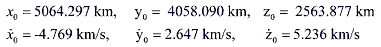(55)

and the drag coefficient was set Cd = 2.635. For the nominal reference trajectory, the initial estimates for the second track estimation were used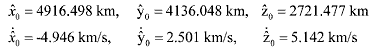(56)

and the initial estimate of the drag coefficient was C^d = 3.0. The process noise covariance matrix used was the same as the first track, except the variance value of the drag coefficient was increased in order to consider uncertainty effects due to the propagation.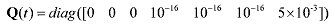(57)

The adaptive nonlinear filters used for the state and parameter estimation are based on the identification of the process noise Q(t). Since each adaptive filter produces a different value of the objective function J that is the sum of the innovation errors, the weight factors calibrated from the Downhill Simplex optimization method are different. The weight factor or wind size γ for the AEKF was 4.5×105, the values for the AUKF and ADDF were approximately equal with γ = 1.65×102.

Figures 4 and 5 are the plots of the performance comparison of the adaptive filters and nonlinear filters with respect to the position and velocity estimation errors in the second track, respectively, which illustrate a possible realistic scenario in orbit determination. From the previous simulation results in the first track, it is expected that the UKF performance should be superior to the EKF performance. However, the UKF results of the averaged magnitudes of the position and velocity estimation errors are very close to those of the EKF with the biased estimation errors. The degradation of the EKF and UKF performance is related to the fact that the state and covariance prediction executed for a long-time interval leads to large prediction errors due to the effects of the neglected nonlinear terms, the parameter uncertainties such as the drag coefficient, and also the initial errors from the 1st track. Thus, the filters start estimating the states with large initial state errors and large covariance matrices with unknown statistical information of the uncertainties or noise. Under the incorrect noise information, even the UKF or higher-order nonlinear filters can’t produce optimal estimates due to the violation of the optimality conditions. On the other hand, all the adaptive filters (AUKF, ADDF and AEKF) converge continuously and fast with small bias error, which indicates they are performing in a near optimal fashion. The performance in the velocity estimation also shows that the adaptive nonlinear filters provide a more accurate estimate than that of the standard nonlinear filters (the EKF and the UKF). This agrees well with our expectations and indicates that the correct noise information is necessary for the filters to perform optimally.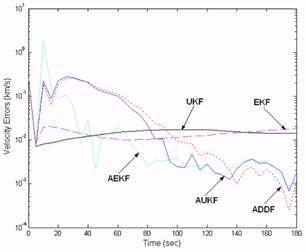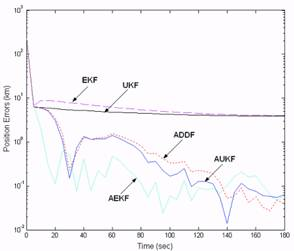Fig. 4. Absolute Position Estimation Error in Second Track. Fig. 5. Absolute Velocity Estimation Errors in Second Track.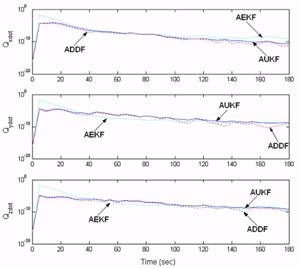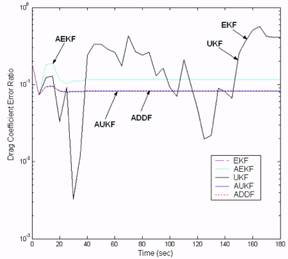Fig. 6. Drag Coefficient Estimation Error Ratio in Second Track. Fig. 7. Adaptive Covariance Estimation with Q Adaptation.

In Fig. 6 the absolute values of the drag coefficient error ratio with respect to the proposed adaptive filters are shown in the available second track, where the error ratio is the ratio between the true and estimated drag coefficient. As expected the parameter estimation with the adaptive filters also successfully generated converged solutions with the fast and accurate estimates. The EKF and UKF also converge, but with large bias errors. In the drag coefficient estimation, the AUKF and ADDF show better performance over the AEKF.

Fig. 7 illustrates the adaptation of the process noise variance generated from the adaptive nonlinear filters. It is seen that while the manually-tuned covariance is constant with time, the estimated covariance has time-varying values that are continuously estimating and adapting the noise statistics for an optimal performance. From the results it is seen that the increased process noise variances at the initial estimation make the prediction covariance and the Kalman gain bigger, therefore the observations have much influence on the filters. In contract as the variances decrease with time, the Kalman gain becomes small and then the effect of the observations on the filters is reduced. For optimal performance of the filters the process noise variance might be required to increase from the initial variances.

Fig. 8 (a)-(c) depicts the innovation errors with the 2-sigma bound. The innovation errors from the adaptive filters vary inside the sigma bound, but the innovations from the EKF and UKF are outside the bound. According to these results, we can also judge that the adaptive filters achieved the near-optimal performance.

According to the criteria of the RMS errors, the nonlinearity index, and the innovation error presented so far, it is seen that the performance of the adaptive nonlinear filters is optimal in the sense that they compensate for the neglected modeling errors as well as the unknown uncertainties.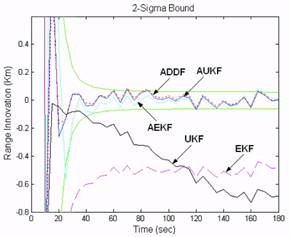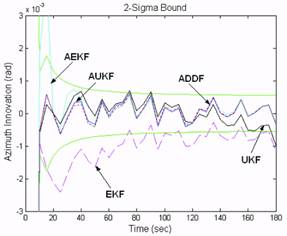Fig. 8-(a). Range Innovation Errors with 2- Sigma Bound. Fig. 8-(b). Azimuth Innovation Errors with 2- Sigma Bound.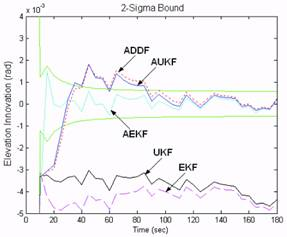Fig. 8-(c). Elevation Innovation Errors with 2-Sigma Bound.
##### 2. Falling Body Tracking Example

In this section the performance of the sigma particle filter algorithm is demonstrated and compared with the nonlinear Gaussian filters including the extended Kalman filter (EKF), the unscented Kalman filter (UKF), and divided difference filter (DDF). To test the performance of the proposed nonlinear filters the vertical falling body example described in Fig. 9 is used because it contains significant nonlinearities in the dynamical and measurement equation. This falling body example originates from Athans, Wishner, and Bertolini , and has become a benchmark to test the performance of newly developed nonlinear filters. For instance, the UKF algorithm  and the DDF  were tested and compared with the Gaussian second-order filter as well as the EKF. See Ref. 25 for additional applications of the nonlinear filters.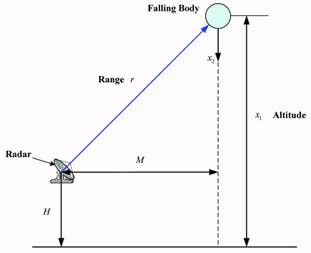Fig. 9. Geometry of the Vertically Falling Body.

The goal of the nonlinear filtering is to estimate the altitude x1, the downward velocity x2, a constant ballistic parameter x3 of a falling body that is reentering the atmosphere at a very high altitude with a very high velocity. The radar measures the range rk which is updated each second and corrupted with additive white Gaussian noise νk which has zero mean and covariance E{νkνkT} = Rk. The trajectory of the body can be described by the first-order differential equations for the dynamic system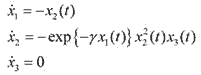(59)

The radar, located at a height H from the mean sea-level and at a horizontal range M from the body, measures the range rk, which is corrupted by Gaussian measurement noise νk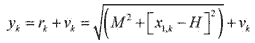(60)

where νk is the measurement noise represented with zero-mean and covariance Rk. The measurements are made at a frequency of 1 Hz. The model parameters are given by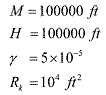(61)

and the initial state x0 of the system is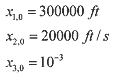(62)

The a priori state estimate x^0 is given by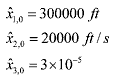(63)

and covariance matrix P0 is selected as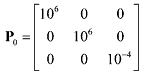(64)

Process noise was not included in the trajectory simulation, therefore Qk = 0. For the numerical integration of the dynamic differential equations small time steps are required due to high nonlinearities of the dynamic and measurement models. A fourth-order Runge-Kutta scheme with 64 steps between each observation is employed to solve the first-order differential equations. For the UKF κ = 0 was chosen in accordance with the heuristic formula na+κ = 3, and h = 3 was used in the DDF. For sigma particle generation in the SPF, the simplified sampling method in Eq. (39) was used. The total number of sigma particles generated was N=91, which is larger than that of the UKF but much smaller than that in the particle filter (usually N is larger than 300 or 1000 in particle filtering). The initial estimates or mean values used in the initial sigma particle sampling step are assumed to be the true values, x0 = x^0. The constant parameter values used for the sigma particle sampler in the SPF were l = 3, τ = 0.1, and 1. The tuning parameters used for the exponentially time-varying measurement noise covariance are λc = 0.023, λs = 10-3, C0 = 0.

The performance of the nonlinear filters is compared in terms of the average root mean square error, which is defined by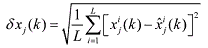(65)

where L is the Monte Carlo simulation run, and subscript j denotes the j-th component of the vector x(k) and its corresponding estimate x^(k), and the superscript i denotes the i-th simulation run. To enable a fair comparison of the estimate produced by each of the four filters, the estimates are averaged by a Monte Carlo simulation consisting of 30 runs.

In Fig. 10 the average magnitude of the position error is depicted by each filter. As can be shown, the performance of the SPF dominates the nonlinear Gaussian filters. The error of the SPF is smaller than the others, and more importantly, the convergence of the estimation of the SPF is much faster than those of the other filters, which is a very encouraging factor for the filter performance. The velocity estimates are shown in Fig. 11 where large peak errors occur in the nonlinear filters, but the SPF recovered quickly and had a smaller peak error. The UKF had smaller errors than the EKF and DDF, but a larger peak error. This spike motion occurred when the radar range was perpendicular to the trajectory and, as such, it didn’t provide enough measurement information to the filters. Fig. 11 shows the SPF can work correctly in a situation where there is not enough measurement information.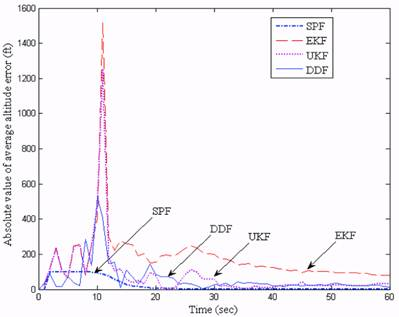Fig. 10. Absolute Errors in Position Estimate.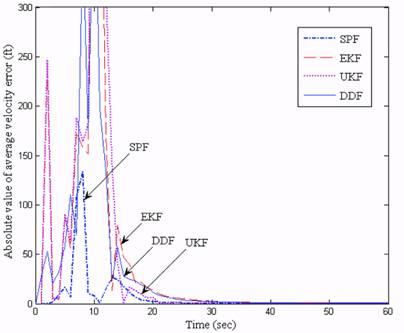Fig. 11. Absolute Errors in Velocity Estimate.

Fig. 12 indicates the errors in estimating the ballistic parameter. The error from the SPF converged quickly to a value very near zero. It shows that the SPF performs better than the EKF and higher-order nonlinear filters. In addition, the performance of the DDF is slightly better than the UKF, but much better than the EKF. The difference of the estimate results between the UKF and DDF is not as dramatic, but they exhibited larger errors than the SPF. In addition, the figures show that the SPF produced nearly unbiased optimal estimates.Fig. 12. Absolute Errors in the Ballistic Coefficient Estimate.
##### CONCLUSIONS

In this paper new nonlinear filtering algorithms called the adaptive unscented Kalman filter, the adaptive divided difference filter, and the sigma particle filter are utilized to obtain accurate and efficient estimates of the state as well as parameters for nonlinear systems with unknown noise statistics. The purpose of the adaptive nonlinear filtering methods is to not only compensate for the nonlinearities neglected by linearization, but also to adaptively estimate the unknown noise statistics for optimal performance of the filters. The sigma particle filtering algorithm can be used for nonlinear and/or non-Gaussain state estimation. Simulation results indicated that the performances of the new nonlinear Bayesian filtering algorithms are superior to the standard nonlinear filters, the extended Kalman filter, the divided difference filter, and the unscented Kalman filter in terms of the estimation accuracy of states and parameters and robustness to uncertainties. In particular, the robustness of the adaptive filters to the unknown covariance information provides the flexibility of implementation without the annoying manual-tuning procedure. In addition, the sigma particle filters can dramatically reduce the computational complexity of a particle filtering implementation by employing an efficient sampling approach called the deterministic sigma particle sampling method. The advantages of the proposed adaptive nonlinear filtering algorithms make these suitable for efficient state and parameter estimation in not only satellite orbit determination, but also other application areas.
##### REFERENCES

1. Jazwinski, A. H., “Adaptive Filtering,” Automatica, Vol. 5, 1969, pp. 475-485.
2. Ho, Y. C., and Lee, R. C. K., “A Bayesian Approach to Problems in Stochastic Estimation and Control,” IEEE Transactions on Automatic Control, Vol. 9, October 1964, pp. 333-339.
3. Anderson, B. D. O., and Moore, J. B., Optimal Filtering, Prentice Hall, Englewood Cliffs, NJ, 1979.
4. Sorenson, H. W., “On the Development of Practical Nonlinear Filters,” Information Sciences, Vol. 7, 1974, pp. 253-270.
5. Kalman, R. E., “A New Approach to Linear Filtering and Prediction Problems,” Transactions on ASME, Journal of Basic Engineering, Vol. 82, March 1960, pp. 34-45.
6. Tanizaki, H., Nonlinear Filters: Estimation and Applications, Springer, New York, 1996.
7. Gelb, A., (ed.), Applied Optimal Estimation, The MIT Press, Cambridge, MA, 1974.
8. Julier, S. J., Uhlmann, J. K., and Durrant-Whyte, H. F., “A New Method for Nonlinear Transformation of Means and Covariances in Filters and Estimators,” IEEE Transaction on Automatic Control, Vol. 45, No.3, March 2000, pp. 477-482.
9. Norgaard, M., Poulsen, N. K., and Ravn, O., “New Developments in State Estimation for Nonlinear Systems,” Automatica, Vol. 36, No. 11, November 2000, pp. 1627-1638.
10. Kastella, K., “Finite Difference Methods for Nonlinear Filtering and Automatic Target Recognition,” in Multitarget-Multisensor Tracking: Applications and Advance, Bar-Shalom, Y., and Blair, W. D., Volume III, Artech House, Norwood, MA, 2000, pp.233-258.
11. Gordon, N. J., Salmon, D. J., and Smith, A. F. M, “A Novel Approach to Nonlinear/Non-Gaussian Bayesian State Estimation,” IEE Proceedings on Radar and Signal Processing, 140, 1993, pp. 107-113.
12. Doucet, A., Godsill, S., and Andrieu, C., “On Sequential Monte Carlo Sampling Methods for Bayesian Filtering,” Statistical Computing, Vol. 10, No. 3, 2000, pp. 197-208.
13. Liu, J. S., Chen, R., “Sequential Monte Carlo Methods for Dynamic Systems,” Journal of the American Statistical Association, Vol. 93, 1998, pp. 1032-1044.
14. Carpenter, J., Clifford, P. and Fearnhead, P., “Improved Particle Filter for Nonlinear Problems,” IEE Proc. Part F: Radar and Sonar Navigation, Vol. 146, February 1999, pp. 2-7.
15. Arulampalam, M. S., Maskell, S., Gordon, N., and Clapp, T, “A Tutorial on Particle Filters for Online Nonlinear/Non-Gaussian Bayesian Tracking,” IEEE Transactions on Signal Processing, Vol. 50, No. 2, Feb. 2002, pp. 174-188.
16. Lee, D.-J., and Alfriend, K. T., “Sigma Point Kalman Filters for Efficient Orbit Estimation,” AAS/AIAA Astrodynamics Specialist Conference, Big Sky, Montana, August 3-7, 2003.
17. Mehra, R. K., “On the Identification of Variances and Adaptive Kalman Filtering,” IEEE Transactions on Automatic Control, Vol. 15, No. 2, April 1970, pp. 175-184.
18. Sage, A. P., and Husa, G. W., “Adaptive Filtering with Unknown Prior Statistics,” 10th Joint Automatic Control Conference, University Memorial Center, University of Colorado, Boulder, Colorado, August 5-7 1969, pp. 760-769.
19. Myer, K. A., and Tapley, B. D., “Adaptive Sequential Estimation with Unknown Noise Statistics,” IEEE Transactions on Automatic Control, Vol. 21, No. 4, August 1976, pp. 520-523.
20. Busse, F. D., and How, J. P., “Demonstration of Adaptive Extended Kalman Filter for Low Earth Orbit Estimation Using CDGPS,” Institute of Navigation GPS Meeting, September 2002.
21. Lee, D.-J., and Alfriend, K. T., “Adaptive Sigma Point Filtering for State and Parameter Estimation,” AIAA/AAS Astrodynamics Specialist Conference, Providence, Rhode Island, August 16-19, 2004.
22. P. Maybeck, Stochastic Models, Estimation, and Control, Vol. 2, Academic Press, New York, 1972.
23. Lee, D.-J., and Alfriend, K. T., “Sigma Particle Filtering for Nonlinear Systems,” AAS/AIAA Astrodynamics Specialist Conference, Lake Tahoe, CA, August 7-11, 2005.
24. Vallado, D. A., Fundamentals of Astrodynamics and Applications, New York, McGraw-Hill, 1997.
25. Athans, M, Wishner, R. P., and Bertolini, A., “Suboptimal State Estimation for Continuous-Time Nonlinear Systems from Discrete Noisy Measurements,” IEEE Transactions on Automatic Control, Vol. 13, No. 3, October 1968, pp. 504-514.
26. Lee, D.-J., Nonlinear Bayesian Filtering with Applications to Estimation and Navigation, Ph.D. Dissertation, Aerospace Engineering Department, Texas A&M University, TX, 2005.

Размещен 24 декабря 2006.